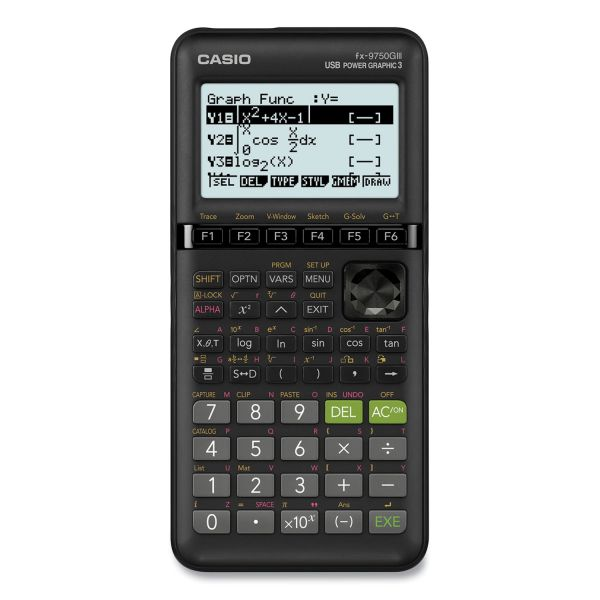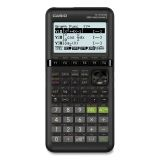# Casio FX-9750GIII 3rd Edition Graphing Calculator, 21-Digit LCD

Product Number: CSOFX9750GIII
• Icon menu provides easy access to advanced functions; Graph rectangular, polar and parametric functions and inequalities, graphical analysis, streamlined solving for intercepts, intersections, etc.
• Python Programming Language and user-selectable order of operation and math input/output.
• 20 graphical functions: Various icon (Run, Table, Dynamic, Recursion and Conics) will allow you to graph or analyze the graphs of given information. You can graph in the form of r = polar coordinates, parametric functions, x =, and y-inequalities.
• The Matrix mode allows you to perform matrix operations on matrices with up to 6 rows and 6 columns.
More details
\$82.07
Free Shipping Estimated Delivery
Favorite
Compare
Request Quote

## Product Description

Casio FX-9750GIII 3rd Edition Graphing Calculator, 21-Digit LCD - The Casio fx-9750GIII 3rd Edition Graphing Calculator supports students and educators from middle school to high school and into college. Its icon-based menu is easy to navigate and the soft-menus make it easy to access functionality for common tasks. The intuitive software design facilitates a student's grasp of mathematical concepts, by encouraging student engagement with mathematics, rather than the memorization of keys to effectively promote student success. Recommended for students taking Pre-Algebra, Algebra I and II, Geometry, Trigonometry, AP Calculus, AP Statistics, Biology, Physics, and Business and Finance.

• Icon menu provides easy access to advanced functions; Graph rectangular, polar and parametric functions and inequalities, graphical analysis, streamlined solving for intercepts, intersections, etc.
• Python Programming Language and user-selectable order of operation and math input/output.
• 20 graphical functions: Various icon (Run, Table, Dynamic, Recursion and Conics) will allow you to graph or analyze the graphs of given information. You can graph in the form of r = polar coordinates, parametric functions, x =, and y-inequalities.
• The Matrix mode allows you to perform matrix operations on matrices with up to 6 rows and 6 columns.
• This calculator includes an icon menu is used to graph parabolas, circles, ellipses, and hyperbolas. You can input a rectangular function, polar coordinate function or a parametric function for graphing.
• Suggested Courses: Pre-Algebra, Algebra I & II, Geometry, Trigonometry, AP Calculus, AP Statistics, Biology, Physics, Business and Finance.
• Dot matrix display 128 x 64.
• Any time while operating the calculator, you can capture an image of the current screen and save it in capture memory to recall at a later time.Guides & Information

### Product Specifications

• +/- Switch Key
No
• Amortization
No
• Assembly Required
No
• Backspace Key
Yes
• Base Number Calculations
No
• Bond Calculations
Yes
• Brand Name
Casio
• Case
Slide-On Hard-Case
• Cash Flow Calculations
No
• Complex Number Calculations
Yes
• Confidence Interval Calculating
No
• Cost/Sell/Margin
No
• Country of Origin
TH
• Currency Exchange Function
No
• Date Calculations
No
• Decimal Function
Yes
• Depreciation Calculations
Yes
• Display Characters Height
4 mm
• Display Characters x Display Lines
21 x 8
• Display Notation
Alphanumeric
• Display Type(s)
LCD
• Display Window Resolution
128 x 64
• Double Zero Key
No
• Entry Logic
V.P.A.M.
• Equation Editor
Yes
• Fraction Calculations
Yes
• Fraction/Decimal Conversions
Yes
• Global Product Type
Calculators-Graphing
• Grand Total Key
No
• Graphing Functions
Rectangular Inequalities Polar Parametric Recursive
• Higher Mathematical Functions
Advanced Statistical Distribution Calculations Derivatives Integrals
• Hyperbolic Functions
Yes
• Hypothesis Testing
Yes
• I/O Port
No
• Interest Rate Conversion
No
• Item Count Function
No
• Levels of Parentheses
26
• Linear Regression
Yes
• Loan Calculation
No
• Logical (Boolean) Operations
No
• Markup/Down Key
No
• Matrices
Yes
• Memory
26 Constant
• Metric Conversion
Yes
• Number of Display Digits
21
No
• Percent Key(s)
No
• Polar-Rectangular Conversion
Yes
• Post-Consumer Recycled Content Percent
0%
• Power Source(s)
Battery
• Pre-Consumer Recycled Content Percent
0%
• Probability (Random Number)
Yes
• Programming Steps
50 Subroutines
• Recycled
No
• Replacement Batteries
AAA
• Simultaneous Equations
Yes
• Size
3.6 x 7.25
• Square Root Key
No
• Tax Calculation
No
• Time-Value-of-Money
Yes
• Time/Date
No
• Total Recycled Content Percent
0%
• Trig/Log Functions
Yes
• Variable Regression
Yes
• Variable Statistics
Yes
• Wall-Mountable
No